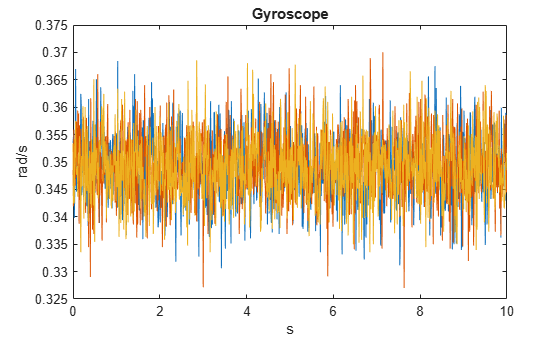# gyroparams

Gyroscope sensor parameters

## Description

The `gyroparams` class creates a gyroscope sensor parameters object. You can use this object to model a gyroscope when simulating an IMU with `imuSensor`. See the Algorithms section of `imuSensor` for details of `gyroparams` modeling.

## Creation

### Syntax

``params = gyroparams``
``params = gyroparams(Name,Value)``

### Description

````params = gyroparams` returns an ideal gyroscope sensor parameters object with default values.```

````params = gyroparams(Name,Value)` configures `gyroparams` object properties using one or more `Name,Value` pair arguments. `Name` is a property name and `Value` is the corresponding value. `Name` must appear inside single quotes (`''`). You can specify several name-value pair arguments in any order as `Name1,Value1,...,NameN,ValueN`. Any unspecified properties take default values.```

## Properties

expand all

Data Types: `single` | `double`

Resolution of sensor measurements in (rad/s)/LSB, specified as a real nonnegative scalar. Here, LSB is the acronym for least significant bit.

Data Types: `single` | `double`

Constant sensor offset bias in rad/s, specified as a real scalar or 3-element row vector. Any scalar input is converted into a real 3-element row vector where each element has the input scalar value.

Data Types: `single` | `double`

Sensor axes skew in percentage, specified as a scalar, a 3-element row vector, or a 3-by-3 matrix. The diagonal elements of the matrix account for the misalignment effects for each axes. The off-diagonal elements account for the cross-axes misalignment effects. The measured state vmeasure is obtained from the true state vtrue via the misalignment matrix as:

`${v}_{measure}=\frac{1}{100}M\text{ }{v}_{true}=\frac{1}{100}\left[\begin{array}{ccc}{m}_{11}& {m}_{12}& {m}_{13}\\ {m}_{21}& {m}_{22}& {m}_{23}\\ {m}_{31}& {m}_{32}& {m}_{33}\end{array}\right]{v}_{true}$`

• If you specify the property as a scalar, then all the off-diagonal elements of the matrix take the value of the specified scalar and all the diagonal elements are 100.

• If you specify the property as a vector [a b c], then m21 = m31 = a, m12 = m32 = b, and m13 = m23 = c. All the diagonal elements are 100.

Data Types: `single` | `double`

Power spectral density of sensor noise in (rad/s)/√Hz, specified as a real scalar or 3-element row vector. This property corresponds to the angle random walk (ARW). Any scalar input is converted into a real 3-element row vector where each element has the input scalar value.

Data Types: `single` | `double`

Filter coefficients for bias instability noise generation, specified as a structure. The structure has these fields:

• `Numerator` — Numerator coefficients, specified as a real-valued vector.

• `Denominator` — Denominator coefficients, specified as a real-valued vector.

To specify coefficients for fractal noise, use the `fractalcoef` function.

Example: `struct(Numerator=1,Denominator=[1 -0.5])`

Data Types: `struct`

Instability of the bias offset in rad/s, specified as a real scalar or 3-element row vector. Any scalar input is converted into a real 3-element row vector where each element has the input scalar value.

Data Types: `single` | `double`

Integrated white noise of sensor in (rad/s)(√Hz), specified as a real scalar or 3-element row vector. Any scalar input is converted into a real 3-element row vector where each element has the input scalar value.

Data Types: `single` | `double`

Type of random noise, specified as:

• `"double-sided"` — Random noise coefficients have a scale factor of 2.

• `"single-sided"` — Random noise coefficients have a scale factor of 1.

Data Types: `char` | `string`

Sensor bias from temperature in ((rad/s)/℃), specified as a real scalar or 3-element row vector. Any scalar input is converted into a real 3-element row vector where each element has the input scalar value.

Data Types: `single` | `double`

Scale factor error from temperature in (%/℃), specified as a real scalar or 3-element row vector with values ranging from 0 to 100. Any scalar input is converted into a real 3-element row vector where each element has the input scalar value.

Data Types: `single` | `double`

Sensor bias from linear acceleration in (rad/s)/(m/s2), specified as a real scalar or 3-element row vector. Any scalar input is converted into a real 3-element row vector where each element has the input scalar value.

Data Types: `single` | `double`

## Examples

collapse all

Generate gyroscope data for an imuSensor object from stationary inputs.

Generate a gyroscope parameter object with a maximum sensor reading of 4.363 $\mathrm{rad}/\mathrm{s}$ and a resolution of 1.332e-4 $\left(\mathrm{rad}/\mathrm{s}\right)/\mathrm{LSB}$. The constant offset bias is 0.349 $\mathrm{rad}/\mathrm{s}$. The sensor has a power spectral density of 8.727e-4 . The bias from temperature is 0.349 $\left(\mathrm{rad}/{\mathrm{s}}^{2}\right)$$/{}^{0}C$. The scale factor error from temperature is 0.2%$/{}^{0}C$. The sensor axes are skewed by 2%. The sensor bias from linear acceleration is 0.178e-3 $\left(rad/s\right)/\left(m/{s}^{2}\right)$

`params = gyroparams('MeasurementRange',4.363,'Resolution',1.332e-04,'ConstantBias',0.349,'NoiseDensity',8.727e-4,'TemperatureBias',0.349,'TemperatureScaleFactor',0.02,'AxesMisalignment',2,'AccelerationBias',0.178e-3);`

Use a sample rate of 100 Hz spaced out over 1000 samples. Create the imuSensor object using the gyroscope parameter object.

```Fs = 100; numSamples = 1000; t = 0:1/Fs:(numSamples-1)/Fs; imu = imuSensor('accel-gyro','SampleRate', Fs, 'Gyroscope', params);```

Generate gyroscope data from the imuSensor object.

```orient = quaternion.ones(numSamples, 1); acc = zeros(numSamples, 3); angvel = zeros(numSamples, 3); [~, gyroData] = imu(acc, angvel, orient);```

Plot the resultant gyroscope data.

```plot(t, gyroData) title('Gyroscope') xlabel('s') ylabel('rad/s')```## Version History

Introduced in R2018b